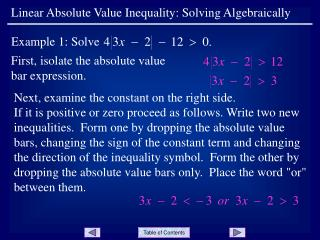DownloadDownload PresentationFirst, isolate the absolute value bar expression.

# First, isolate the absolute value bar expression.

Télécharger la présentation## First, isolate the absolute value bar expression.

- - - - - - - - - - - - - - - - - - - - - - - - - - - E N D - - - - - - - - - - - - - - - - - - - - - - - - - - -
##### Presentation Transcript

1. Example 1: Solve Linear Absolute Value Inequality: Solving Algebraically First, isolate the absolute value bar expression. Next, examine the constant on the right side. If it is positive or zero proceed as follows. Write two new inequalities. Form one by dropping the absolute value bars, changing the sign of the constant term and changing the direction of the inequality symbol. Form the other by dropping the absolute value bars only. Place the word "or" between them.

2. The solution set in interval notation is Linear Absolute Value Inequality: Solving Algebraically Now solve each inequality. The "or" means that any number that satisfies either of the inequalities will be a solution of the original inequality. Notes: If after isolating the absolute value expression the constant term on the right is negative, the solution set of the inequality would be (- , ) because any real number substituted for x will cause the absolute value expression to produce a number greater than a negative number. Slide 2

3. Example 2: Solve Linear Absolute Value Inequality: Solving Algebraically First, isolate the absolute value bar expression. Next, examine the constant on the right side. If it is positive or zero proceed as follows. Write two new inequalities. Form one by dropping the absolute value bars, changing the sign of the constant term and changing the direction of the inequality symbol. Form the other by dropping the absolute value bars only. Place the word "and" between them. Slide 3

4. The solution set in interval notation is Linear Absolute Value Inequality: Solving Algebraically Now solve each inequality. The "and" means that only those numbers that satisfy both of the inequalities will be solutions of the original inequality. Notes: If after isolating the absolute value expression the constant term on the right is negative, the inequality would have no solutions because no real number substituted for x will cause the absolute value expression to produce a number less than a negative number. Slide 4

5. Linear Absolute Value Inequality: Solving Algebraically END OF PRESENTATION Click to rerun the slideshow.